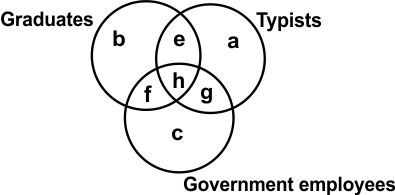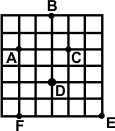﻿ Reasoning Olympiad (CRO) Sample Paper & Questions for Class 10

# CREST Reasoning Olympiad Class 10 Sample Paper

Sample PDF of CREST Reasoning Olympiad for Class 10:

## Syllabus:

Section 1: Series Completion, Analogy and Classification, Coding - Decoding, Blood Relations, Sequential Output Tracing , Direction Sense Test, Logical Venn Diagrams, Alphabet Test and Logical, Alpha-Numeric, Number, Mathematical Operations, Inserting the Missing Characters, Analytical Reasoning, Mirror Images and Water Images, Spotting Out The Embedded, Figure Matrix, Paper Folding and Paper Cutting, Grouping of Identical Figures, Cubes and Dice, Dot Situation, Construction of Squares and Triangles, Figure Formation and Analysis.

Achievers Section: Higher Order Thinking Questions - Syllabus as per Section 1

 Q.1 If '+' means '-', '÷' means '+', '-' means '×' and '×' means '÷', then which of the following equation is correct?
 Q.2 Shweta starts from point A and covers 4 km in North-East direction to reach point B, then she turns towards South-East and covers another 4 km to reach point C. Now, she turns towards South-West and travels another 4 km to reach point D. Finally, she turns towards North-West and travels another 4 km to reach point E. Calculate the shortest distance between points A and E.
 Q.3 Study the following information carefully to answer the question. 'A \$ B' means 'A is the mother of B'. 'A # B‘ means 'A is the father of B'. 'A @ B' means 'A is the husband of B'. 'A % B' means 'A is the daughter of B'. If F@D%K#H, then how is F related to H?
 Q.4 Which set of letters completes the second pair in the same way as the first pair? ADJM is to ZWQN, as FKIM is to ___________.
 Q.5 The figure given below is made of three circles intersecting one another. These circles represent graduates, typists and government employees. The intersecting regions have been denoted by a, b, c, e, f, g and h, respectively. Study the diagram carefully and answer the question that follows. Which of the following letters represents the typists are only graduates?Q.6 Study the following map and answer the question. Here, A, B, C, D, E and F are six cities. Cities A and F are to the ________ of city B.Q.7 Study the given relationship and complete the following analogies from the two sets of options given. Square is to (i), as Circle is to (ii)(i) [A] Rectangle [B] Cube [C] Cuboid [O] Triangle(ii) [W] Ellipse [X] Ellipsoid [Y] Sphere [X] Line
 Q.8 At an office where an interview was conducted to select persons for clerical posts, they came to know that, out of 20 persons, 12 knew only typing and 5 knew only shorthand and the rest knew both typing and shorthand. Which of the following diagrams represents this fact?
 Q.9 In this question, relationship between different elements is shown in the statements. The statements are followed by two conclusions. Statements: A > B, B = H, H > GConclusions: I. A > G, II. A > H
 Q.10 Amongst four friends Sara, Betty, Georgia and Cathy, Betty is taller than Cathy but not taller than Sara, Georgia's height is shorter than that of Sara. Which of the following is not possible?

 Q.1 c Q.2 d Q.3 a Q.4 b Q.5 a Q.6 b Q.7 b Q.8 c Q.9 c Q.10 d

If you are getting prepared for the CREST Reasoning Olympiad exam the CRO sample papers become extremely helpful.

The CREST Reasoning Olympiad previous years’ question papers for class 10 can assist students in comprehending the exam pattern and format. Students can prepare for the exam by using the CRO sample question paper. Through sample question papers, students can practice & improve their preparation for the exam. Students can use the sample question papers for class 10 CRO to continue improving their Reasoning skills and logical thinking abilities.

Here you can find the most recent sample papers for the class 10 CREST Reasoning Olympiad exam (CRO). Download a free PDF of the Reasoning Olympiad sample paper for class 10 to give you a head start for your exam.